# MetaTrader 5 与 Python 的集成：接收和发送数据

3 四月 2019, 07:18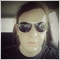5
6 284

### 利用 Python 创建套接字服务器

```import socket, numpy as np
from sklearn.linear_model import LinearRegression
```

```class socketserver:
def __init__(self, address = '', port = 9090):
self.sock = socket.socket(socket.AF_INET, socket.SOCK_STREAM)
self.port = port
self.cummdata = ''

def recvmsg(self):
self.sock.listen(1)
self.cummdata = ''

while True:
data = self.conn.recv(10000)
self.cummdata+=data.decode("utf-8")
if not data:
break
self.conn.send(bytes(calcregr(self.cummdata), "utf-8"))
return self.cummdata

def __del__(self):
self.sock.close()
```

recvmsg 方法监听传入连接 sock.listen(1)。 当传入的客户端连接到达时，服务器会接受它 self.sock.accept()。

```def calcregr(msg = ''):
chartdata = np.fromstring(msg, dtype=float, sep= ' ')
Y = np.array(chartdata).reshape(-1,1)
X = np.array(np.arange(len(chartdata))).reshape(-1,1)

lr = LinearRegression()
lr.fit(X, Y)
Y_pred = lr.predict(X)
type(Y_pred)
P = Y_pred.astype(str).item(-1) + ' ' + Y_pred.astype(str).item(0)
print(P)
return str(P)
```

```serv = socketserver('127.0.0.1', 9090)

while True:
msg = serv.recvmsg()
```

### 利用 MQL5 创建套接字客户端

socksend() 函数将数据传递给服务器：

```bool socksend(int sock,string request)
{
char req[];
int  len=StringToCharArray(request,req)-1;
if(len<0) return(false);
return(SocketSend(sock,req,len)==len);
}
```

```string socketreceive(int sock,int timeout)
{
char rsp[];
string result="";
uint len;
uint timeout_check=GetTickCount()+timeout;
do
{
if(len)
{
int rsp_len;
if(rsp_len>0)
{
result+=CharArrayToString(rsp,0,rsp_len);
}
}
}
while((GetTickCount()<timeout_check) && !IsStopped());
return result;
}
```

```void drawlr(string points)
{
string res[];
StringSplit(points,' ',res);

if(ArraySize(res)==2)
{
Print(StringToDouble(res));
Print(StringToDouble(res));
datetime temp[];
CopyTime(Symbol(),Period(),TimeCurrent(),lrlenght,temp);
ObjectCreate(0,"regrline",OBJ_TREND,0,TimeCurrent(),NormalizeDouble(StringToDouble(res),_Digits),temp,NormalizeDouble(StringToDouble(res),_Digits));
}

```

```void OnTick() {
socket=SocketCreate();
if(socket!=INVALID_HANDLE) {
if(SocketConnect(socket,"localhost",9090,1000)) {
Print("Connected to "," localhost",":",9090);

double clpr[];
int copyed = CopyClose(_Symbol,PERIOD_CURRENT,0,lrlenght,clpr);

string tosend;
for(int i=0;i<ArraySize(clpr);i++) tosend+=(string)clpr[i]+" ";
drawlr(recieved); }

else Print("Connection ","localhost",":",9090," error ",GetLastError());
SocketClose(socket); }
else Print("Socket creation error ",GetLastError()); }
```

### 测试 MQL5-Python 客户端 - 服务器应用程序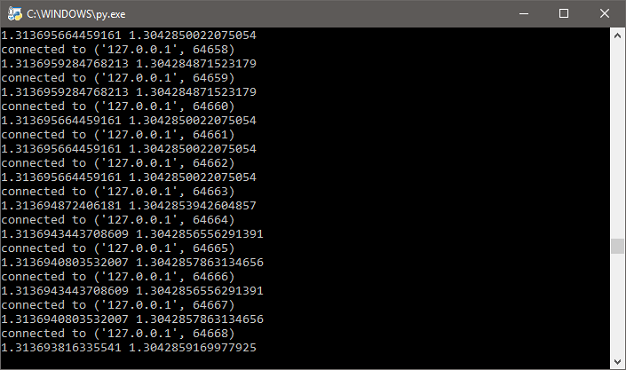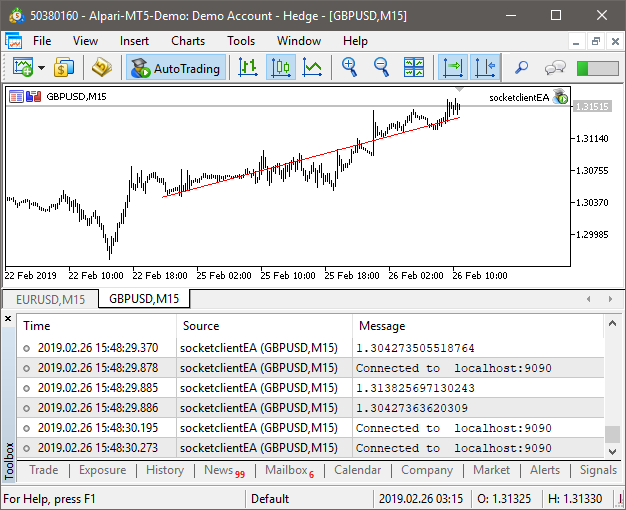### 使用 MetaTrader 5 Python API 获取并分析报价

```pip install MetaTrader5
```

```from MetaTrader5 import *
from datetime import date
import pandas as pd
import matplotlib.pyplot as plt

# 初始化 MT5 连接
MT5Initialize()
MT5WaitForTerminal()

print(MT5TerminalInfo())
print(MT5Version())
```

```# 为需要绘制相关矩阵的货币创建清单
sym = ['EURUSD','GBPUSD','USDJPY','USDCHF','AUDUSD','GBPJPY']

# 将数据复制到数据帧
d = pd.DataFrame()
for i in sym:
rates = MT5CopyRatesFromPos(i, MT5_TIMEFRAME_M1, 0, 1000)
d[i] = [y.close for y in rates]
```

```# 逆初始化 MT5 连接
MT5Shutdown()

# 比较百分比变化
rets = d.pct_change()

# 计算相关性
corr = rets.corr()

# 绘制相关性矩阵
plt.figure(figsize=(10, 10))
plt.imshow(corr, cmap='RdYlGn', interpolation='none', aspect='auto')
plt.colorbar()
plt.xticks(range(len(corr)), corr.columns, rotation='vertical')
plt.yticks(range(len(corr)), corr.columns);
plt.suptitle('FOREX Correlations Heat Map', fontsize=15, fontweight='bold')
plt.show()
``````# 导入 statsmodels 进行协整检验
import statsmodels
from statsmodels.tsa.stattools import coint

x = d['GBPUSD']
y = d['GBPJPY']
x = (x-min(x))/(max(x)-min(x))
y = (y-min(y))/(max(y)-min(y))

score = coint(x, y)
print('t-statistic: ', score, ' p-value: ', score)
```

```# 绘制 z-分值变换
diff_series = (x - y)
zscore = (diff_series - diff_series.mean()) / diff_series.std()

plt.plot(zscore)
plt.axhline(2.0, color='red', linestyle='--')
plt.axhline(-2.0, color='green', linestyle='--')

plt.show()
```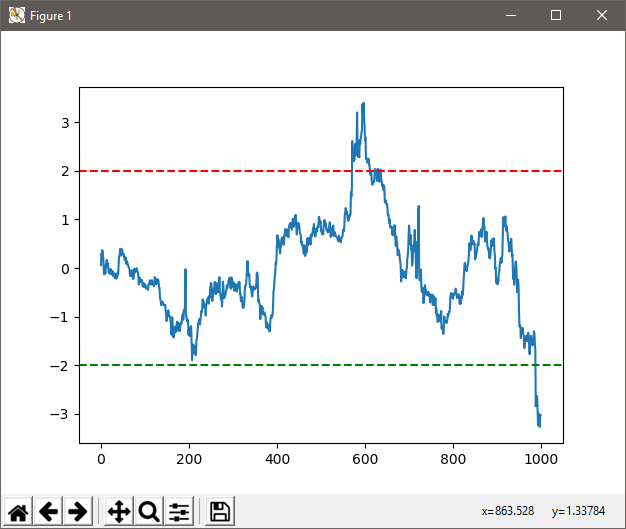### 利用 Plotly 函数库可视化市场数据

```# -*- coding: utf-8 -*-
"""

@author: dmitrievsky
"""
from datetime import datetime
import pandas as pd
# 初始化 MT5 连接
MT5Initialize()
MT5WaitForTerminal()

print(MT5TerminalInfo())
print(MT5Version())

# 将数据复制到 pandas 数据帧
stockdata = pd.DataFrame()
rates = MT5CopyRatesFromPos("EURUSD", MT5_TIMEFRAME_M1, 0, 5000)
# 逆初始化 MT5 连接
MT5Shutdown()

stockdata['Open'] = [y.open for y in rates]
stockdata['Close'] = [y.close for y in rates]
stockdata['High'] = [y.high for y in rates]
stockdata['Low'] = [y.low for y in rates]
stockdata['Date'] = [y.time for y in rates]

import plotly.graph_objs as go

trace = go.Ohlc(x=stockdata['Date'],
open=stockdata['Open'],
high=stockdata['High'],
low=stockdata['Low'],
close=stockdata['Close'])

data = [trace]
plot(data)
```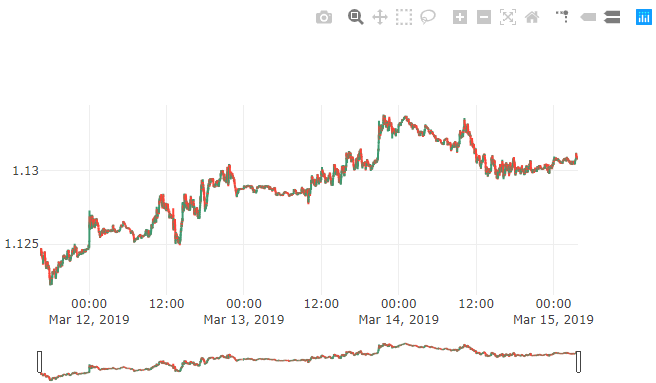```# -*- coding: utf-8 -*-
"""

@author: dmitrievsky
"""
from datetime import datetime

# 初始化 MT5 连接
MT5Initialize()
MT5WaitForTerminal()

print(MT5TerminalInfo())
print(MT5Version())

# 将数据复制到列表
rates = MT5CopyTicksFrom("EURUSD", datetime(2019,3,14,13), 1000, MT5_COPY_TICKS_ALL)
bid = [x.bid for x in rates]
time = [x.time for x in rates]

# 逆初始化 MT5 连接
MT5Shutdown()

import plotly.graph_objs as go
data = [go.Scatter(x=time, y=bid), go.Scatter(x=time, y=ask)]

plot(data)
```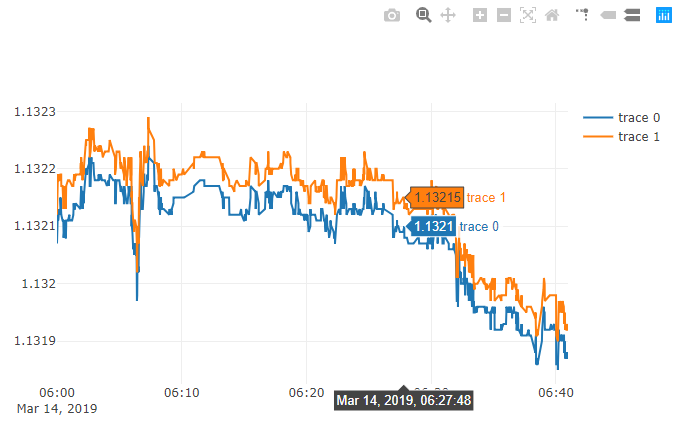### 结束语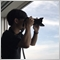| 10 4月 2019 在 10:29
MT5终于和python对接了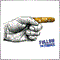| 10 4月 2019 在 14:20
Bohao Zhang:
MT5终于和python对接了| 10 7月 2019 在 06:41| 10 7月 2019 在 10:12
chenjianzhe:

Hi, sockets don't work in tester at this time. Also, you mus add localhost to allowed webrequests in mt5 settings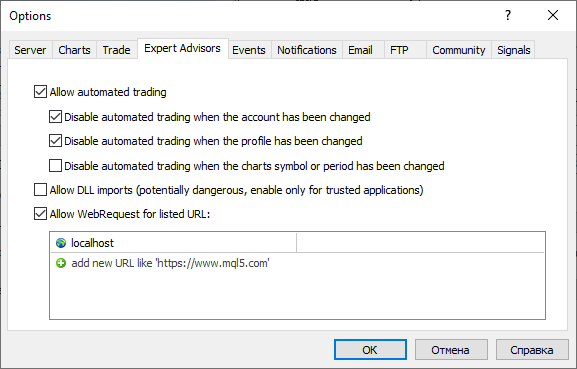| 11 9月 2019 在 17:46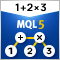利用MQL进行MQL解析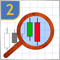研究烛条分析技术(第二部分)：自动搜索新形态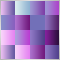交易策略的色彩优化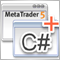基于 .Net 框架和 C# 为 EA 交易和指标开发图形界面